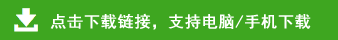# 小学二年级上册数学期中检测卷

小学二年级上册数学期中检测卷+参考答案部分内容预览

一、填一填。(15分)
1.18÷6=(    ),读作:(　),其中被除数是(    ),除数是(    ),商是(    )。
2. 8+8+8+8+8+8=8×(    )　9+9=(    )×2
3.一只手有5根手指,两只手有(    )根手指,两双手有(    )根手指。
4.两个乘数都是6,积是(    );两个加数都是5,和是(    )。
5. 5个4相加的和比17多(    );6个6比70少(    )。
6.有十几个小朋友在玩游戏,把他们平均分成4组,每组人数一样多,最多有(    )个小朋友。
7.把6+6+6+6+6-10改写成乘减算式是(　)。
二、判一判。(对的在括号里画“√”,错的画“ ”)(8分)
1.笔算两位数加法,从高位加起。(    )
2. 2×5读作2乘5;6÷2读作6除以2。(    )
3. 2+2可以写成2×2;3+3可以写成3×3。(    )
4.把8个☆分给4个小朋友,每人一定有2个。(    )
三、把口诀补充完整。(9分)
二三(　)　 四(    )二十四　三四(　)
三六(　)    五五(　)         (    )六三十六
(    )二得四    (　)十六       (　)得一
四、做一做。(31分)
1.直接写得数。(5分)
6×5=　16÷4=　  15÷3=　4×4=　5×3-5=
9÷3=     5×4=    1×6=     3×4+1=   4×6-7=
2.选一选。(6分)
平行四边形有(    )。
3.列竖式计算。(12分)
70-24-16=       28+36+19=
71-34+26=      64+17-25=
4.在下面的方格纸上画一个平行四边形。(3分)
5.哪些分法是平均分?在括号里画“√”。(5分)